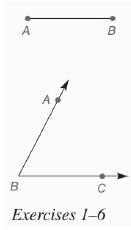Chapter 7.CR, Problem 6CRElementary Geometry For College St...

7th Edition
Alexander + 2 others
ISBN: 9781337614085

Solutions

Chapter
SectionElementary Geometry For College St...

7th Edition
Alexander + 2 others
ISBN: 9781337614085
Textbook Problem

In Review Exercises 1 to 6, use the figure shown.Construct a rhombus with side A B ¯ and ∠ A B C .To determine

To construct: A rhombus with one side of length AB and ABC.

Explanation

First, draw line segment AB and construct angle BAX = 60 degrees.

With A as the center and radius equal to length AB, draw an arc on ray AX. Mark the point of intersection as D and with D as the center and radius equal to length AB, draw an arc C.

Still sussing out bartleby?

Check out a sample textbook solution.

See a sample solution

The Solution to Your Study Problems

Bartleby provides explanations to thousands of textbook problems written by our experts, many with advanced degrees!

Get Started

Find more solutions based on key concepts